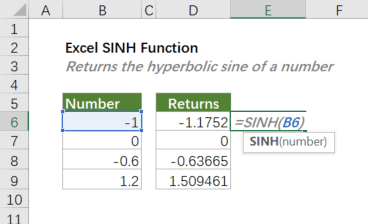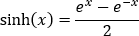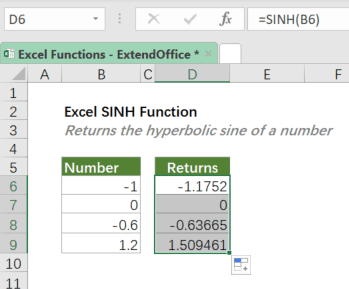Note: The other languages of the website are Google-translated. Back to English

## Excel SINH Function

The SINH function calculates the hyperbolic sine of a number.=SINH(number)

#### Arguments

• number (required): The angle in radians for which you want to calculate the hyperbolic sine.

#### Return Value

The SINH function returns a numeric value.

#### Function Notes

• SINH returns the #VALUE! error if number is not numeric.
• The formula for the hyperbolic sine is:#### Example

To calculate the hyperbolic sine of the numbers listed in the table as shown below, please copy or enter the below formula in the top cell (D6) of the result list, and press Enter to get the result. Then select the result cell, and drag the fill handle (the small square in the lower-right corner of the selected cell) down to apply the formula to the below cells.

=SINH(B6)Instead of a cell reference, you can type the actual number value in the formula as shown below.

=SINH(-1)

#### Related functions

Excel SIN Function

The SIN function calculates the sine of an angle in radians.

Excel ACOSH Function

The ACOSH function returns inverse hyperbolic cosine of a number.

Excel ACOTH Function

The ACOTH function returns the inverse hyperbolic cotangent of a number.

Excel ASINH Function

The ASINH function returns the inverse hyperbolic sine of a number.

Excel ATANH Function

The ATANH function returns the inverse hyperbolic tangent of a number.

Excel COSH Function

The COSH function returns the hyperbolic cosine of a number.

Excel COTH Function

The COTH function returns the hyperbolic cotangent of a hyperbolic angle.

Excel CSCH Function

The CSCH function returns the hyperbolic cosecant of an angle specified in radians.

Excel SECH Function

The SECH function calculates the hyperbolic secant of an angle in radians.

Excel TANH Function

The TANH function calculates the hyperbolic tangent of a number.

### The Best Office Productivity Tools

#### Kutools for Excel - Helps You To Stand Out From Crowd

Would you like to complete your daily work quickly and perfectly? Kutools for Excel brings 300 powerful advanced features (Combine workbooks, sum by color, split cell contents, convert date, and so on...) and save 80% time for you.

• Designed for 1500 work scenarios, helps you solve 80% Excel problems.
• Reduce thousands of keyboard and mouse clicks every day, relieve your tired eyes and hands.
• Become an Excel expert in 3 minutes. No longer need to remember any painful formulas and VBA codes.
• 30-day unlimited free trial. 60-day money back guarantee. Free upgrade and support for 2 years.#### Office Tab - Enable Tabbed Reading and Editing in Microsoft Office (include Excel)

• One second to switch between dozens of open documents!
• Reduce hundreds of mouse clicks for you every day, say goodbye to mouse hand.
• Increases your productivity by 50% when viewing and editing multiple documents.
• Brings Efficient Tabs to Office (include Excel), Just Like Chrome, Firefox, And New Internet Explorer.